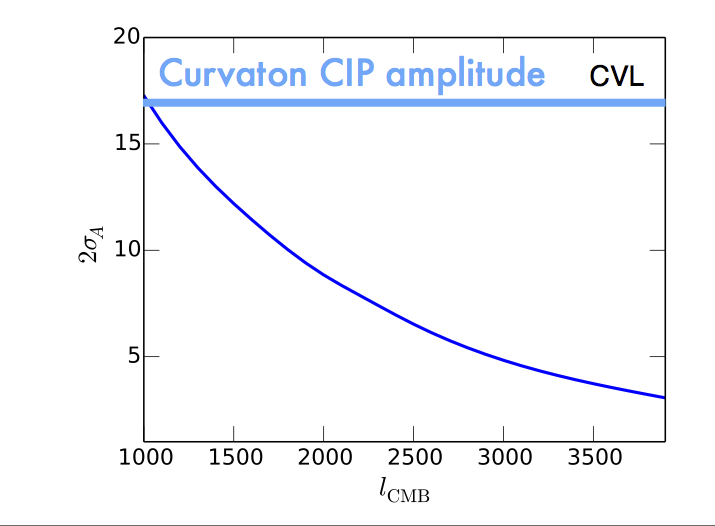# Compensated isocurvature perturbations in the curvaton model

Compensated isocurvature perturbations (CIPs) are fluctuations in the relative density of baryons and cold dark matter (CDM) at fixed total matter density, which are detectable using CMB data as a result of a spatially varying sound speed in the baryon-photon plasma. In this work, graduate student Chen He (mentored by me & supervised by Prof. Wayne Hu) assessed the detectability of CIPs produced by a spectator field during inflation, called a curvaton. These CIPs would accompany the usual adiabatic mode. We found that a CMB Stage-IV experiment could detect such CIPs. This project was a great opportunity to mentor Chen, who did most of the hard work (picture below).Results published in arXiv:1505.00639 (PhysRev. D 92.063018).

Collaborators: Chen He  & Wayne Hu.

A CIP (shown by the cartoon on top) can be generated in the curvaton model while respecting CMB limits to the total isocurvature amplitude, if baryon number and cold dark matter are generated by/before curvaton decay, respectively (or vice-versa). The resulting CIP is proportional to the primordial curvature perturbation$\zeta$), since it is also sourced by quantum fluctuations in the curvaton field during the inflationary era. The CIP amplitude is set by the curvaton fraction at decay,$r_{\rm D}$. By correlating the resulting correlations between different CMB multipole moments to reconstruct a CIP map$\Delta(\hat{n})$) and then correlating this map with the CMB map yet again (a procedure then dominated by large scale primary CMB anisotropies), one can test the curvaton model. Below we show the threshold for a$2\sigma$ detection of CIPs, where A relates the CIP amplitude to the curvature fluctuation via the relation$\Delta(\hat{n})=A \zeta$. The results are shown below, as a function of the smallest angular scale (highest multipole moment)$l_{\rm max}$ for which primary CMB anisotropies can be detected in a cosmic-variance limited experiment (the planned CMB Stage-IV experiment should approach this limit). There are claims that small-scale polarized foregrounds can be effectively removed to much higher scales than temperature anisotropies, so we show results as a function of$l_{\rm max}$ .